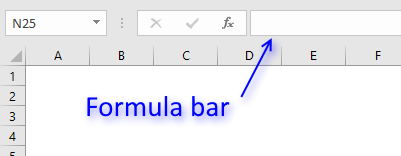Author: Oscar Cronquist Article last updated on January 15, 2019

This user defined function creates a unique distinct list of words and how many times they occur in the selected range.

### User defined Function Syntax

FREQWORDS(cell_range, position)

### Arguments

 cell_range Required. The range you want to use. position Required. Which column to return. The first column contains the values and the second column contains their corresponding frequency.

### Example

Array formula in cell E3:E30:

=FreqWords(B2:C11, 1)

Array formula in cell F3:F30:

=FreqWords(B2:C11, 2)

How to create an array formula

1. Select cell range E3:E30.
2. Copy (Ctrl + c) array formula and paste (Ctrl + v) to formula bar.3. Press and hold Ctrl + Shift simultaneously.
4. Press Enter once.
5. Release all keys.

### VBA

```'Name function and declare arguments
Function FreqWords(tbl_array As Range, pos As Integer) As Variant()
'Declare variables and their data types
Dim cell As Variant, wrds As Variant, i As Integer
Dim a As Integer, j As Integer
Dim tmp() As String, nr() As Integer
'Redimension variable tmp so it can grow using Redim Preserve
ReDim tmp(0), nr(0)

'Assign 1 to first value in array variable nr
nr(0) = 1
'Iterate through cells in cell range
For Each cell In tbl_array
'Split words in cell
wrds = Split(cell)
'Iterate thorugh words
For i = 0 To UBound(wrds)
'Iterate through arrayvariable tmp
For j = 0 To UBound(tmp)
'If variable wrds equal variable tmp then increase value in variable nr by 1
If wrds(i) = tmp(j) Then
nr(j) = nr(j) + 1
a = 1
Exit For
End If
Next j
'Check if a is not equal to 1
If a <> 1 Then
'Copy value from variable wrds to tmp
tmp(UBound(tmp)) = wrds(i)
'Add another container to array variable tmp
ReDim Preserve tmp(UBound(tmp) + 1)
ReDim Preserve nr(UBound(tmp))
nr(UBound(tmp)) = 1
End If
a = 0
Next i
Next cell
'Return values in column 1 if argument pos is equal to 1
If pos = 1 Then
ReDim Preserve tmp(UBound(tmp) - 1)
FreqWords = Application.Transpose(tmp)
Else
'Return values in column 2 if argument pos is not equal to 1
ReDim Preserve nr(UBound(nr) - 1)
FreqWords = Application.Transpose(nr)
End If
End Function
```

### How to add the User defined Function to your workbook

1. Press Alt-F11 to open visual basic editor
2. Click Module on the Insert menu
3. Copy and paste vba code
4. Exit visual basic editor